Latest SSC jobs   »   Mathematics Quiz For RRB NTPC :...

# Mathematics Quiz For RRB NTPC : 18th December

Q1. The ratio of the income of Ram and Shyam is 7 : 17 and the ratio of the income of Shyam and Sohan is 7 : 17. If the income of Ram is Rs. 490 then what is the income of Sohan ?
(a) Rs. 1690
(b) Rs. 2890
(c) Rs. 1790
(d) Rs. 1190

Q2.Mohan and Sohan started a business. Mohan invested Rs. 20,000 for 6 months. Sohan invested some amount for one year. At the end of a year Mohan got Rs. 6,000 in the total profit of Rs. 9,000. How much did Sohan invest initially?
(a) Rs. 10,000
(b) Rs. 5,000
(c) Rs. 12,000
(d) Rs. 8,000

Q3. The average age of Ram and his two children is 17 years and the average age of Ram’s wife and the same children is 16 years. If the age of Ram is 33 years, the age of his wife is(in years):
(a) 31
(b) 32
(c) 35
(d) 30

Q4. Out of 10 teachers of school, one teacher retires and in his place, a new teacher of age 25 years joins. As a result, average age of teachers is reduced by 3 years. The age (in years) of the retired teacher is:
(a) 58
(b) 60
(c) 55
(d) 50

Q5. What will be the difference in compound interest and simple interest of Rs. 40,000 at 5% per annum in 3 years?
(a) 300
(b) 205
(c) 305
(d) 200

Q6. A man invested of his capital at 7%, at 8% and the remainder at 10%. If his annual income is Rs. 561, the capital is
(a) 5400
(b) 6000
(c) 6600
(d) 7200

Q7. A shopkeeper marks the price of an article at Rs 80. What will be the selling price, if he allows two successive discounts at 5% each?
(a) Rs 72.2
(b) Rs 72
(c) Rs 85
(d) Rs 7.2

Q8. Which of the following successive discounts is better to a customer-
(a) 20%, 15%, 10%
(b) 25%, 12%, 8%
(a) (a) is better
(b) (b) is better
(c) (a) or (b) (both are same)
(d) None of these

Q9. The respective ratio of boys and girls in a college is 31 : 23. After the admission of 75 more girls in the college, this ratio becomes 124 : 107. How many girls will have to admit in the class to make the number of boys and girls equal in the college ?
(a) 75
(b) 90
(c) 60
(d) 85

Q10. The sum of present ages of A and B is 7 times the difference of their ages. 5 years hence, their total ages will be 9 times the difference of their ages. What is the present age of elder one (in years) ?
(a) 25
(b) 20
(c) 15
(d) 18

Solutions:

S1. Ans. (b)
Sol. Ram : Shyam : Sohan = 49 : 119 : 289

S2. Ans. (b);
Sol.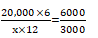=Rs. 5000

S3. Ans. (d)
Sol.
Total age of ram + 2 children = 17 × 3 = 51 year
Total age of both the children = 51- 33 = 18 yr
Total age of ram’s wife + both the children = 16 × 3 = 48 yr
Required age of Ram’s wife = 48 – 18 = 30 yr

S4. Ans, (c)
Sol.
Let the initial average of age = x
Let the retired teacher’s age = T
∴ 10x – T + 25 = 10 (x – 3)
10x – T + 25 = 10x – 30
-T + 25 = -30
T = 55

S5. Ans. (c)
Sol. Direct formula , D = (〖P.r〗^2 (300+r))/〖(100)〗^3 = 305

S6. Ans. (c)
Sol.S7. Ans. (a)
Sol. Successive discount of 5% = 9.75%
9.75% of 80 = 7.8
So, S.P. = 80 – 7.8 = 72.2 Rs.

S8. Ans. (b)
Sol. successive discount of 20%, 15%, 10% = 38.8%
& successive discount of 25%, 12%, 8% = 39.28%
clearly seen, (2) deal is better.

S9. Ans. (d)
Sol.
B G
31 : 23
124 : 107
75 girl – add., but boys remain same.
So, 31 × 4 = 124
124 : 92

124 : 107

15 Ratio = 75
1 Ratio = 5
Diff. = 124-107
So, Req. No. = 17 × 5 = 85

S10. Ans. (b)
Sol. x + y = 7 (x-y) or 6x – 8y = 0 _______(i)
x + 5 + y + 5 = 9 (x – y)
8x-10y = 10 _________ (ii)
After solving (i) & (ii)
x = 20 year
y = 15 year

#### Congratulations!

General Awareness & Science Capsule PDF

Incorrect details? Fill the form again here

General Awareness & Science Capsule PDF

Thank You, Your details have been submitted we will get back to you.
•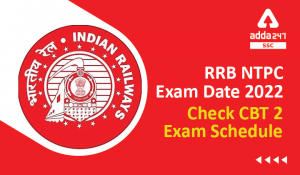RRB NTPC Exam Date 2022 Out for CBT 2, C...
•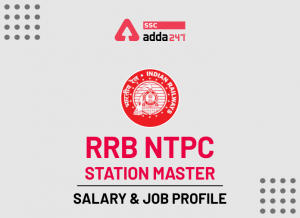Railway Station Master Salary, Job Profi...
•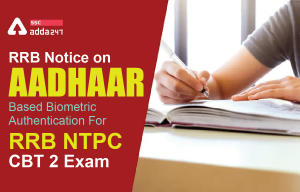RRB Notice on AADHAAR Based Biometric Au...
•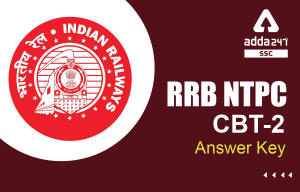RRB NTPC CBT 2 Answer Key 2022 Out, Rais...
•RRB NTPC CBT 2 Cut Off: Check Region Wis...
•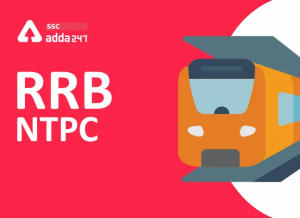RRB NTPC CBT 2 Admit Card 2022 Notificat...